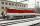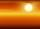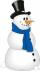# Temperature + reason - math problems

#### Number of problems found: 5

• Ground levelThe temperature at ground level in Helsinki is 20°C lower than in London. The temperature in a basement flat in Helsinki is 5°C below the temperature at ground level. If it is -29°c in the basement, what is the temperature in London?
• Warmer weatherThis morning it was -6 °C. What temperature did the thermometer show yesterday if it was three times warmer?
• Trains for peopleIt is said that the train is synonymous to delay. Calculate the average speed of travel by train long 85 km, with regular train leave at 7:00 and arrive at 8:18, but train is late and has departure at 8:10 and arrive at 9:27.
• Extreme temperaturesUSA hit extreme heat wave. Calculate percentage of the air temperature change from normal summer temperature 23 °C to 40 °C.
• Temperature calculusCalculate the percentage of temperature increase if changed from 18°C to 33°C?

We apologize, but in this category are not a lot of examples.
Do you have an exciting math question or word problem that you can't solve? Ask a question or post a math problem, and we can try to solve it.

We will send a solution to your e-mail address. Solved examples are also published here. Please enter the e-mail correctly and check whether you don't have a full mailbox.

Temperature - math problems. Reason - math problems.